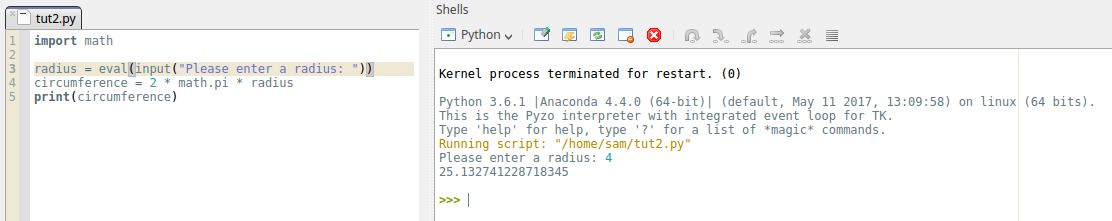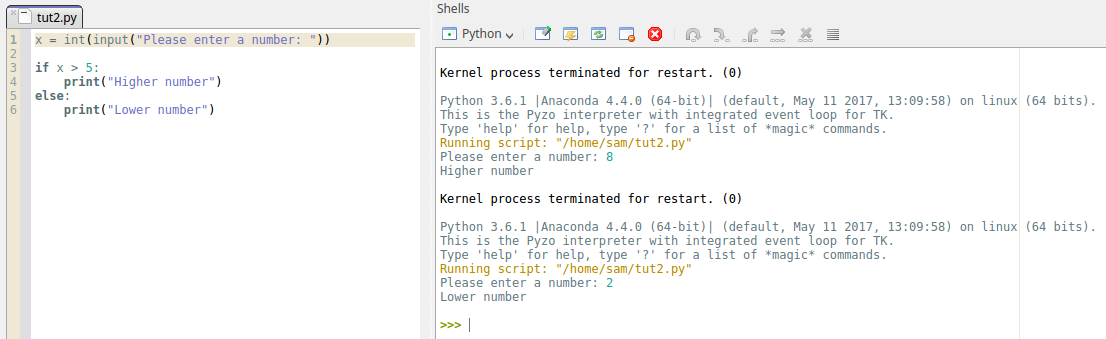# Working With Data Types In Python

It doesn’t matter what programming language you use they will all include data types. These data types are an integral part of how programming languages work so it’s important to understand what each of them are and what they do.

#### What are data types?

Data types are used to define what a piece of data is. If the data is numerical, the interpreter or compiler needs to know this before you can work with it to avoid potential errors. Programming languages that require variables to be used according to their data types are known as strongly typed. Languages that allow variables of one data type to be used as if they are variables of another data type are known as weakly typed.

#### Pythons data types

Python, the language we will be looking at in this article, has four data types. These data types allow the Python interpreter to know how the programmer intends to use a piece of data.

Here are the four data types used in Python.

int - Integers are whole numbers and can be both positive or negative. In some languages integers are defined as short or long depending on how much data storage is used for the number. This isn’t the case in Python though as all whole numbers are simply classed as integers.

float - These are numbers that have a decimal point. In other languages these can sometimes be referred to as decimals or doubles but Python keeps it simple and just uses float. Float is short for floating point which means you can control where the decimal point is placed. Double on the other hand refers to the amount of storage needed for the number.

str - Strings are made of characters and digits that are written inside of quotation marks. A good way to think of a string is as plain text. It doesn’t matter if the string is made of letters or numbers, the interpreter views it as text because the data is wrapped inside of quotation marks. This is also the reason why you can have spaces in strings. Without the quotation marks, the interpreter would view each word as separate causing a whole host of problems. These quotation marks are a clever way to group text together, allowing for easier manipulation.

bool - Boolean values are true or false values. They are used in expressions to determine what the program should do next, based on whether something is true or false.

Here are some examples of how these look when assigned to variables in Python.

x = 5

This is an example of an int. It shows that the variable “x” has been assigned the value of 5 which is an integer.

y = 4.17

This is an example of a float. As you can see “y” has been assigned the value of 4.17, a floating point number.

z = “Hello”

This is an example of a str. You can see that “z” has been assigned the value of a string because the data is inside quotation marks.

b = True

Finally, this is an example of a bool. In this example, variable “b” has been assigned the value of True. It’s important to note the capital “T” in the word True. Without it, Python wouldn’t recognise it as a boolean value and the same rule applies to False as well.

#### How do you use them?

So, now you understand the basics of data types, let’s start working with them. Below I have written some basic programs to help you understand how different types work.

Below is a simple program for calculating the circumference of a circle based on its radius.This programs first asks a user for the size of the radius. The word int in this program automatically converts any input to an integer. This avoids a lot of potential errors with the input. The input message itself is a string. As you can see from the shell, the user has entered 4. The radius variable now has the value of 4.

Now the program can execute the next line of code. The equation is now performed which gives us the circumference, and this value is assigned to the circumference variable. Finally the last line of code executes and prints the value of the circumference variable which gives the user the answer.

Although rather basic, this gives you an example of how data types are often used when writing real programs. Now we will write another program to show how boolean values are used. This program will use an if-statement. This is a statement that tells the program to execute a certain piece of code if the expression is true. Here is the example.This program asks the user for a number then uses an if-statement to tell the user whether the number is higher or lower than 5. Now let's look at the if-statement itself. The top part is the boolean expression. It’s saying that if the expression x > 5 is true, execute the next line of code. The first number entered was 8 which is greater than 5, so the program returned the output “Higher number”.

If the expression is false the program moves to the “else” statement and then executes the code beneath that instead. The second number entered was 2 so the program executed the else statement, printing “Lower number” instead. This is a pretty useless program but it serves a purpose for this demonstration.

#### What next?

It’s worth going over this material till you have a solid idea of how all of these data types work. Create your own short programs and figure out for yourself what these data types can and can’t do. Trial and error is one of the best ways to learn programming and spending the time going over the basics will really help later on when you start writing the more complex stuff.

If you feel confident already and want to move onto something more involved, if-statements are a good place to start. They are probably the easiest programming concept to understand and once you know how to use them, they open a lot of doors in terms of what you can do with your programs.